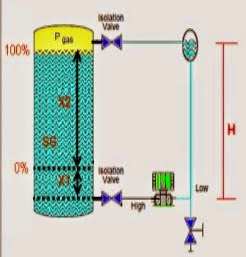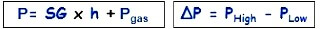# Closed tank DP Level Transmitter with wet leg elevation zero direct mount Calibration

The DP Level transmitter installed very near to HP tapping point and configured in wet leg.Schematic Diagram of a Closed tank DP Level Transmitter with wet leg elevation zero direct mount CalibrationClosed tank DP Level Transmitter with wet leg elevation zero direct mount CalibrationFormulas:

Min range = (SG x X1) – (SG x H)

Max range = [(SGxX1) + (SGxX2)] – (SGxH)

Example:

If the height is 0.5 cashew an X1, X2 is 2 meters and height H is 3 meters, there is fluid in the tank which has a density of 1 g / cm3, what is the range of calibration on the transmitter?

SG = 1

Min range = P High – P Low

= (SG x X1) – (SG x H)
= (1 x 0.5) – (1 x 3)
= 0.5 to 3
= – 2.5 mH 2 O

Max range = P High – P Low

= [(SGxX1) + (SGxX2)] – (SGxH)
= [(1×0,5) + (1×2) – (1×3)
= 2.5 – 3
= -0.5 MH 2 O

The transmitter Range = -2.5 to -0.5 mH 2 O

Don't Miss Our Updates
Be the first to get exclusive content straight to your email.
We promise not to spam you. You can unsubscribe at any time.
1.